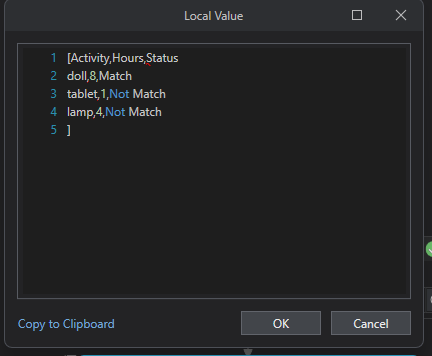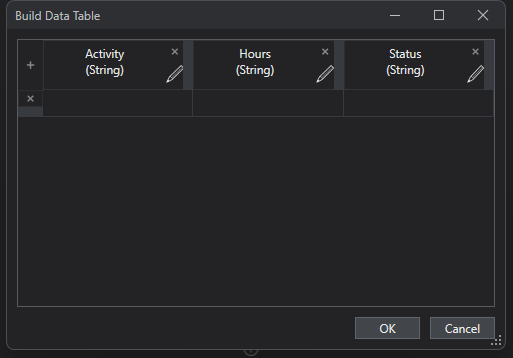# Compare 2 different columns and the output in another column using linq query

Hi all,

Linq.xlsx (8.6 KB)
Sheet 1 consists of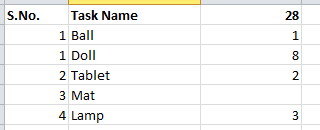Sheet 2 consists of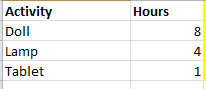Need to compare the Task name and Activity with 28 and Hours columns and the result should be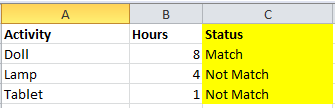If the task name and hours are not same then need to give not match else match need to give.

try below way

2. add data column for the second table
3. Use invoke code activity and refer below thread to find the linq query

Finally write the datatable to excel

Regards

1 Like

find the file

testlookup.xaml (8.7 KB)

``````For Each row1 As DataRow In dt1.Rows
For Each row2 As DataRow In dt2.Rows
If row1("Activity").ToString() = row2("Task Name").ToString() AndAlso row1("Hours").ToString() = row2("28").ToString() Then
row1("Status") = "Matched"
Exit For
Else
row1("Status") = "Not matched"
End If
Next
Next
``````

Assuming Status column will be available other have to use add data column activity before invoke code

Regards

1 Like

Initialize dtResult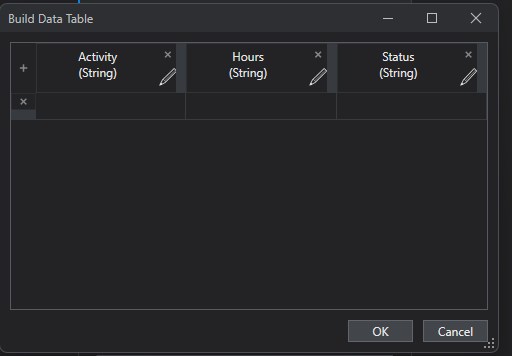Then read first excel as dtInput and read excel 2 as dt2
and then just filter

``````(From row In dtInput
Join row2 In dt2
Let hours = If(row("Hours").ToString() = row2("Hours").ToString(), "Match", "Not Match")
Let ra = New Object(){row("Task Name"), row2("Hours"), hours}